Courses

# Job Costing & Batch Costing - Methods of Costing, Cost Accounting B Com Notes | EduRev

## B Com : Job Costing & Batch Costing - Methods of Costing, Cost Accounting B Com Notes | EduRev

The document Job Costing & Batch Costing - Methods of Costing, Cost Accounting B Com Notes | EduRev is a part of the B Com Course Cost Accounting.
All you need of B Com at this link: B Com

Job Costing
It means ascertaining costs of an individual job, work order or project separately. According to ICMA London, “job costing is that form of specific order costing which applies where work is undertaken to customer’s specific requirements and each order is of comparatively of short duration.” Under this method of costing, each job is considered to be a distinct cost unit. As such, each job is separately identifiable.
In the case of a job, work is usually carried out within the factory or workshop. Sometimes, a job is accomplished even in the customer’s premises. This method of costing is applicable to ship building, printing, engineering, machine tools, readymade garments, shoes, hats, furniture, musical instruments, interior decorations etc.

Features:

1. Each job has its own characteristics, depending up on the special order placed by the customer.
2. Each job is treated as a cost unit.
3. A separate job cost sheet is made out for each job on the basis of distinguishing numbers.
4. A separate work in progress ledger is maintained for each job.
5. The duration of the job is normally a short period.
6. Profit or loss is determined for each job independently of others

1. It helps to distinguish profitable jobs from unprofitable jobs
2. It helps to identify defective work and spoilage with a department or person
3. Selling price of special orders can easily be fixed.
4. It helps to prepare estimates of cost for submitting quotations and tender for similar jobs
5. It helps to control future cost.

Requisites of Job costing system:

1. A sound system of production control
2. An effective time booking system
3. Clearly defined cost centre
4. Appropriate overhead absorption rate, and
5. Proper material issue pricing method.

Procedure for Job order costing system:
The Procedure for job order costing system may be summarized as follows:-

1. Receiving an enquiry from the customer regarding price, quality etc
2. Make an estimation of the price of the job after considering the cost incurred for the execution of similar job in the previous year
3. Receiving an order, if the customer is satisfied with the quotation price and other terms of execution.
4. If the job is accepted, a production order is made by the Planning department.
5. The costs are collected and recorded for each job under separate production order Number, and a Job Cost Sheet is maintained for that purpose.
6. On completion of job, a completion report is sent to costing department.

Illustration I
From the following particulars calculate the cost of Job No.505 and price for the job to give a profit of 25% on the selling price.
Material : Rs. 6820
Wage details:
Department X : 60 hrs @ Rs. 3 per hr
Y : 50 hrs @ Rs. 3 per hr
Z : 30 hrs @ Rs. 5 per hr
The variable Overheads are as follows:
Department X : Rs. 5000 for 5000 hrs
Y : Rs. 4000 for 2000 hrs
Z : Rs. 2000 for 500 hrs
The total fixed expenses amounted to Rs. 20,000 for 10,000 working hours
Calculate the cost of Job No. 505 and price for the job to give a profit of 25% on selling price
Solution:

 Job Cost Sheet No. 505 Rs. Direct Material 6,820 Wages: Department X 60 x 3 =180 Department Y 50 x 3 =150 Department Z 30 x 5 =150 480 Prime Cost 7,300 Overheads: - Variables Department X 60 x 1 = 60 Department Y 50 x 2 = 100 Department Y 30 x 4= 120 280 7,580 Fixed OH 140 x 2 = 280(60+50+30 x 2) 280 Total cost 7,860 Profit 25% on selling price ie 1/3 of cost 7860 x 1/3 2, 620 Selling price 10,480

Batch Costing
This is another form of job costing which is adopted in case of manufacturing of a large number of components of machines or of other articles. Since a large number of them are manufactured together and pass through the same process of manufacture, it is convenient to collect their cost of manufacture together. Separate job cost sheets are maintained for each batch of products. Each batch is allotted a number. Material requisitions are prepared batchwise, the direct labour is engaged batchwise and the overheads are also recovered batchwise. Cost of each component in the batch is then determined by dividing the total cost by the number of articles manufactured.

Feature of Batch Costing
Features of batch costing system are as under:

• Batch costing is applied in industries where identical products are produced.
• A batch is a cost unit which consists of a separate, readily identifiable group of product units which maintains its separate identity throughout the production process.
• The output of batch consists of a number of units and it is not economical to ascertain cost of every unit of output independently
• The procedure is very similar to job costing:

(a) Each batch is treated a job and costs are calculated for total batch.
(b) On completion of production cost per unit is found as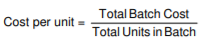Difference between Job Costing and Batch Costing

 Job Costing Batch Costing It is carried out or a product is produced by specific orders. The process of producing the product has a continuous flow and product is homogeneous. It is determined for each job. It is compiled on time basis. Each job is separate and independent of other jobs. Product lose their individuality as they are manufactured in a continuous flow

Applications of Batch Costing
Batch costing is used for calculating total cost of each batch. Batch is small group of units which is produced for production purposes. We also identify batch of units in our production. All raw material is supplied on batch basis and other expenses are also paid on the basis of each batch.
For instance, in the drugs industry, producer will make the batch of tablets instead of producing single tablet. This will be easy to sell that batch in market. So, calculating cost of each batch, we will calculate material cost per batch, labour cost per batch and other expenses per batch. If we want to calculate cost per unit, we have to divide total batch cost with total batch units.
It is used in following industries:

2. Manufacturing industry for toys
3. Manufacturing industry for tyre & tube

Batch Costing Problem 1:
ABC Limited manufactures ring binders which are embossed with the customers’ own logo. A customer has ordered a batch of 600 binders. The following illustrate the cost for a typical batch of 100 binders.

 Rs Direct materials 60 Direct labour 20 Machine set up 6 Design and art work 30 Prime cost 116

Direct employees are paid on a piecework basis.
ABC Limited absorbs production overheads at a rate of 20% of direct wages cost. 5 % is added to the total production cost of each batch to allow for selling, distribution and administration overheads.
ABC Limited requires a profit margin of 25% of sales value.
The selling price for 600 binders (to the nearest penny) will be:
A. Rs 756
B. Rs 772.8
C. Rs 806.4
D. Rs 1008
Solution.

 Rs Prime cost (Rs116 x 6) 696 Overheads (` 20 x 6 x 20%) 24 720 Selling, distribution and admin overheads (180 x 5%) 36 Total cost 756 Selling price (756/75x100) 1008

Batch Costing Problem 2:
Find out the Economic Batch Quantity from the particulars given below:
Annual demand Rs.9,600
Setting up Cost Rs.200
Cost of production per unit Rs.50
Rate of interest 10% p.a.
Solution.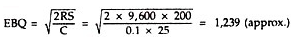Batch Costing Problem 3:
A Contractor has to supply 10,000 paper cones per day to a Textile unit. He finds that when he starts a production run he can produce 25,000 paper cones per day. The cost of holding a paper cone in stock for one year is 2 paise and the set up cost of a production run is Rs.18. How frequently the production run be made:
Solution.
(i) Based on 300 days in the year.
Annual Sales = 10,000 x 300 = 30,00,000 Paper Cones.
Set up Cost = Rs.18
Carrying Cost = Re. 0.02 per unit p.a.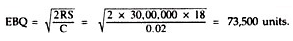73,500 + 10,000 = 7.35
So, Production run should be made in 7 or 8 working days.

Batch Costing Problem 4:
B K Bearing Ltd. is committed to supply 24,000 bearings per annum to Century Fans Ltd. on a steady daily basis. It is estimated that it costs 10 paise as inventory holding cost per bearing per month and that the set-up cost per run of bearing manufacture is Rs.324:
(i) What should be the optimum run size for bearing manufacture?
(ii) What should be the interval between two consecutive optimum runs?
(iii) Assuming that the company has a policy of manufacturing 6,000 bearings per run, how much extra costs the company would be incurring as compared to the optimum run suggested in (i) above?
(iv) Find out the minimum inventory holding cost.
Solution.
(i) Optimum run size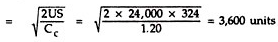where U = units to be produced in a year
S = set-up cost
Cc = carrying cost per unit for one year (12 x 0.10 = Rs. 120)
No. of runs = 24,000 20 /3,600 = 20/ 3
(ii) Interval between two consecutive optimum runs :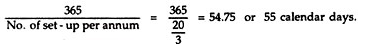Example and Solution.
Illustration 1:
Batch No. A-100 incurred the following costs: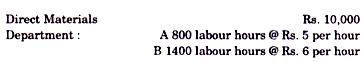Factory overheads are absorbed on labour hours basis and rates are Rs 7per hour for Department A and Rs 4 per hour for Department B. The firm uses a cost plus system for selling prices and expects a 25%gross profit (sales value min us factory cost). Administrative overheads are absorbed at 10% selling price. Assuming that 1000 were units produced in Batch A-100. Calculate the selling prices per units.
Solution.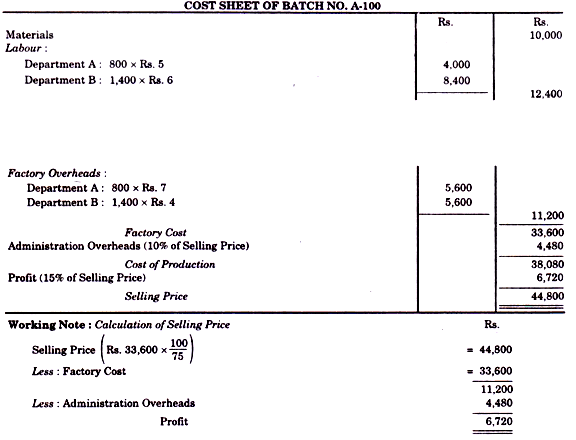Illustration 2:
Compute the economic batch quantity for a company using batch costing with the following information: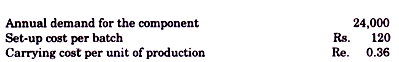Solution.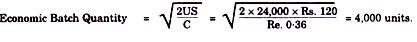Illustration 3:
The annual demand of a product is 24,000 units. It is produced in batches and the largest size of a single batch is 6,000 units. After each batch is complete, the set up cost is Rs. 750. The annual carrying cost is Rs. 2.25 per unit.
Assume average inventory as one-half of the number of units made in each batch. Selecting 4, 6, 8, 12 and 24 batches per annum, determine annual costs of each and state the optimum number of batches to minimize the total costs.
Solution.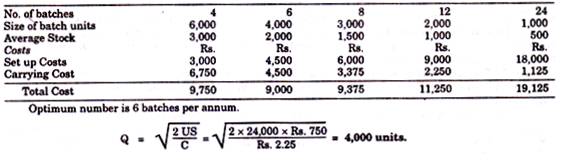Illustration 4:
The demand of an item is uniform at a rate of 25 units p.m. The set up cost is Rs. 30 each time a production is made. The production cost is Rs. 3 per item and the inventory carrying cost is 50 paise per unit p.m. If the shortage cost is Rs. 3 per item p.m. determine how often to make a production run and of what size? Also calculate re-order level.
Solution.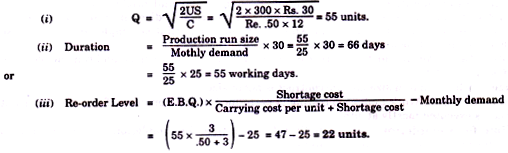Offer running on EduRev: Apply code STAYHOME200 to get INR 200 off on our premium plan EduRev Infinity!

## Cost Accounting

131 videos|139 docs

,

,

,

,

,

,

,

,

,

,

,

,

,

,

,

,

,

,

,

,

,

,

,

,

;